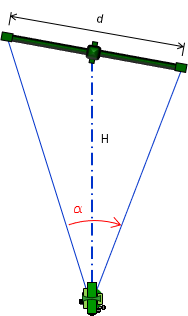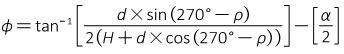## 3. Errors

Although subtense bars are past technology, discussion of their major errors provides some insight on their use.

### a. Instrumental

#### (1) Geometry

##### (a) Principle

The triangle formed by the subtense bar and instrument is a long narrow isosceles triangle. The angle measured at the instrument is small making for some weak geometry, particularly in long distances.

For example, most subtense bars were 2 meters long and transits/theodolites typically had angle measurement resolutions between 6" to 20". Solving Equation D-1 backwards means in a distance of 100 meters, the angle at the instrument is 1°08'45". An angular error of 15" changes the distance by 0.36 meters, an distance error of approximately 1/200.

Random.

##### (c) Compensation

Appropriate measuring instrument, repeated measurements, shorter distances.

#### (2) Orientation

##### (a) Principle

Distance determination is predicated on the subtense bar being perpendicular to the line. Most had a sighting system which helped orient the bar correctly. However, any orientation error, Figure C-5, would affect the basic geometry.Figure C-5Orientation
##### (b) Behavior

Misorientation of the bar introduces a systematic error, Figure C-6.Figure C-6Misorientation Effect

Figure D-6 uses a short slope distance, S, to more clearly show the geometry. The incorrect angle, β, is measured instead of the correct one, α. Using β in equation D-1 will introduce an error in the slope distance. Although systematic, unless the rotation angle, ρ, is known, the horizontal angle can't be corrected. Because of the rotation, the incorrect angle isn't symmetric about the slope distance. Equations C-5 and C-6 are the corrections applied to β for the left rotation of Figure D-6; they are slightly different for a right rotation.Equation C-5Equation C-4

Without knowing the rotation angle and its direction, however, it's impossible compute the corrections. Even if the rotation were known, the horizontal angle corrections would be small. For example, at 100 meters, a 2 meter long bar rotated 10° results in a horizontal angle error of 0°00'07". This would be well inside pointing and setup errors.

So even though the error is systematic, its behavior is random.

##### (c) Compensation

Experience; Careful equipment set up.

### b. Natural

#### (1) Refraction and Curvature

##### (a) Principle

The line of sight, on which subtense bar use depends, is bent by the atmosphere and deviates from a level line due to earth curvature. The effect of each increases as does distance. However, the line of sight to each end of the bar deviates the same amount and in the vertical direction so the combined effect does not affect the horizontal angle measurement.

Is it significant enough to affect the zenith/vertical angle? Refer to Table H-1 in I. Basic Principles Chapter H. Curvature and Refraction:

 Table H-1 Distance α; seconds C; feet 100 0.5 0.000 200 1.0 0.001 300 1.5 0.002 400 2.0 0.004 500 2.5 0.006 1000 4.9 0.024 2000 9.9 0.096 4000 19.7 0.383 5000 24.7 0.598

At a distance of 1000 ft (pushing it with a subtense bar) and a 65°00'00" zenith angle (pretty steep), varying the angle 4.9" makes a difference of 0.01 ft horizontal and 0.02 ft vertical, neither of which affects the distances.

For all intents and purposes, curvature and refraction don't affect subtense bar measurements.

##### (b) Behavior

Systematic but negligible.

Not necessary

#### (2) Atmospheric conditions

##### (a) Principle

In addition to refraction, local atmospheric anomalies can have varying effect when the line of sight passes near a radiant surface which reflects direct sunlight pr emits absorbed heat. The line of sight in such conditions is not stable which introduces pointing errors.

Random

##### (c) Compensation

Select locations either removed from heat wave sources or limit observations to time of day (early) before the sun heats the intermediate surface or absorption takes place.

#### (3) Temperature

##### (a) Principle

Subtense bars are constructed of low thermal expansion alloy materials. They are not affected by temperature changes sufficiently to alter distance measurement within useful operating range.

Temperature is more an instrument operator comfort issue.

##### (b) Behavior

Systematic but negligible.

Not necessary.

### c. Personal

#### (1) Set up

##### (a) Principle

Both the subtense bar and measuring instrument bust be set up over their respective points. This is more fully discussed in the Electronic Distance Measurement section.

Random

##### (c) Compensation

Careful set ups with practice will minimize the random error.

#### (2) Pointing

##### (a) Principle

The surveyor must sight both targets as accurately as possible. Longer distances are increasingly affected as the overall angle between subtense target gets smaller.

Random

##### (c) Compensation

Careful field procedures; Experience; Repeat angles

Mistake

Due diligence.